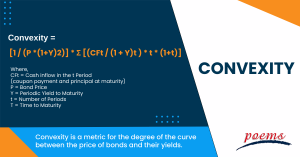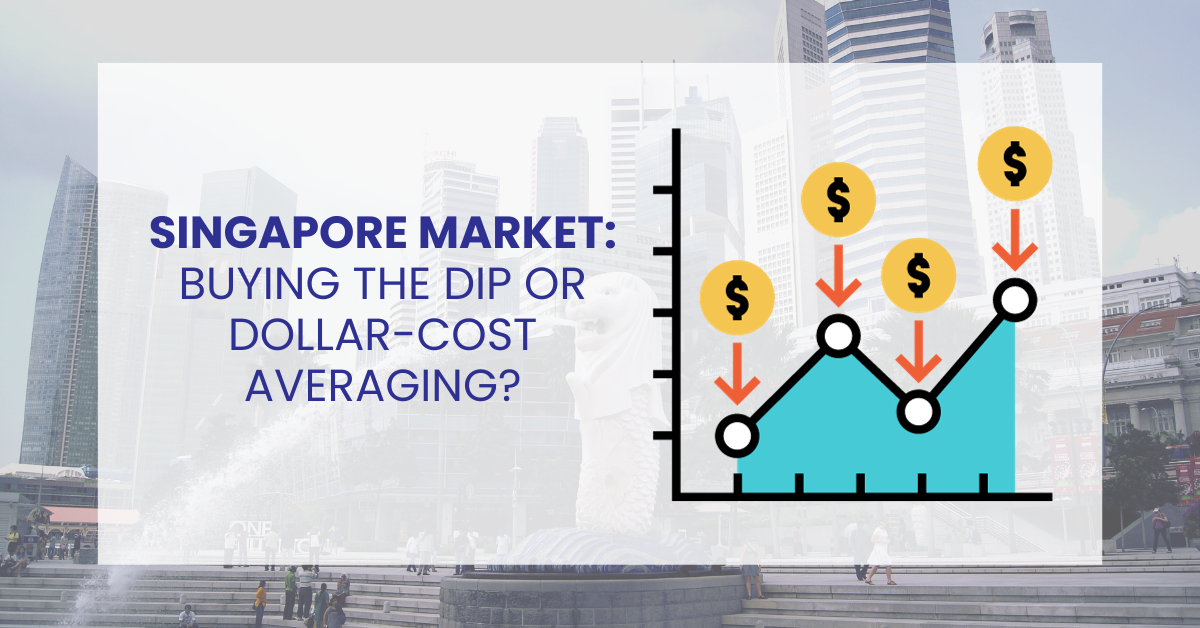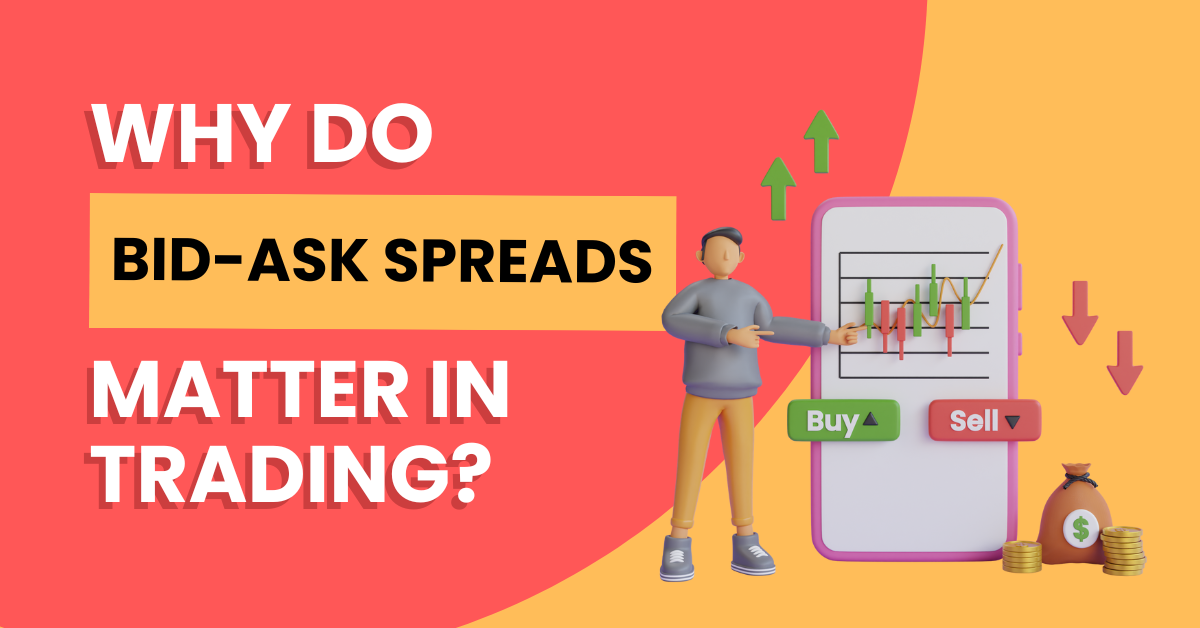﻿ Bond Convexity: What is it, duration, negative & positive, Example, FAQ

# Convexity

## Convexity

Applying the duration and convexity ideas is crucial when assessing bonds. A bond’s or bond portfolio’s approximate price change can be predicted quite well using duration. However, duration loses accuracy in projecting price movements for assets with embedded options and bigger yield changes. Duration and convexity can be coupled to approximate the price for a given change in yield more closely.

The convexity of a bond portfolio can significantly impact the portfolio’s overall return. For example, a portfolio with positive convexity will typically outperform a portfolio with negative convexity when interest rates rise. This is because the YTM curve of a portfolio with positive convexity will become steeper as interest rates rise, which means that the portfolio’s overall return will increase faster than a portfolio with negative convexity.

Overall, the convexity of a bond portfolio is an important consideration for investors when choosing which bonds to include in their portfolio.## What is convexity?

Convexity is a metric for the degree of the curve between the price of bonds and their yields. Thus, convexity is a metric for the arc of the bond price–interest rate connection. It displays how quickly a bond’s duration alters in response to changes in interest rates.

Convexity shows how the term of a bond changes when the interest rate changes. If a bond’s duration increases along with yields, this is called negative convexity. If a bond’s tenure lengthens while its yield drops, it is said to have positive convexity.

## Understanding convexity

Convexity and duration are two calculated metrics that, unfortunately for bond investors, are difficult to get. Although you could theoretically calculate it on your own in Excel, and professional fund managers use sources like Bloomberg to seek this information, your best bet is to find a broker. This is because it isn’t worth the effort to learn the formula, alter it, and use it in Excel regularly. If your broker doesn’t have a bond calculator, his fixed-income offerings may be inadequate.

On a portfolio level, convexity is also incredibly helpful. When managing a bond portfolio, you can utilize duration and convexity to choose where to allocate existing bond positions and new purchases based on the portfolio’s duration and convexity.

Apply the same interest-rate forecast criterion when considering the overall impact of a new position and its allocation. Using position sizes and diversity to reduce risk, you can still buy bonds you like, even if their duration or convexity is at odds with your predicted interest rate.

## Convexity and duration

Investors can learn how interest rate changes will impact a bond’s price using duration and convexity measures. The course of a bond can help investors comprehend the implications for a bond’s price should interest rates change by indicating how a bond’s price responds to changes in interest rates. A bond’s convexity can be used to calculate the difference in duration for a specific change in yields.

If rates are anticipated to rise, consider investing in bonds with shorter periods. These bonds will see less market volatility and less sensitivity to yield increases than bonds with longer periods. Higher-term bonds should be taken into consideration if rates are predicted to fall. Higher-duration bonds stand to gain more than their lower-duration counterparts when rates fall, and bond prices rise.

## Positive and negative convexity

If a bond’s duration increases while the yield decreases, this is referred to as positive convexity. An increase in price brought on by a decrease in rates will have a greater impact on a bond with positive convexity than a rise in price brought on by an increase in yields.

Negative convexity happens when the tenure of a bond increases at the same time that the yield does. Bond prices will fall as the yield increases. When interest rates fall, bond prices rise, but a bond with negative convexity depreciates as rates fall.

## Example of convexity

Consider XYZ Corporation, a bond issuer, as having two bonds on the market now: bond A and B. Each bond has a 100,000 USD face value and a 5% coupon rate. Bond B matures in 10 years as opposed to bond A’s 5-year maturity. Bond A has a period of 4 years, but bond B has a duration of 5.5 years using the concept of duration. This implies that the price of bond A will vary by 4% for every 1% change in interest rates, whereas the price of bond B will change by 5.5%.

Let’s now assume that interest rates unexpectedly rise by 2%. Accordingly, the cost of bond A should go down by 8%, while the cost of bond B would go down by 11%. However, based solely on bond B’s term, the price movement will be less than anticipated using the idea of convexity.

This is because bond B has a higher convexity. After all, it has a longer maturity. Bond B’s higher convexity protects against changes in interest rates, resulting in a less significant price movement than predicted based on tenure.

Convexity = [1 / (P *(1+Y)2)] * Σ [(CFt / (1 + Y)t ) * t * (1+t)]

Where,

CFt = Cash inflow in the t Period (coupon payment and principal at maturity)

P = Bond Price

Y = Periodic Yield to Maturity

t = Number of Periods

T = Time to Maturity

The convexity of a bond gauges how sensitive its duration is to variations in yield. A bond’s price change can’t be accurately measured by duration since it suggests that the change is linear when it has a sloped or “convex” shape.

The bond convexity formula is computed by dividing the total of the bond’s discounted future cash inflow by the number of years it will be outstanding by the sum of the bond’s discounted future cash inflow.

We include the convexity adjustment to our initial duration calculation to estimate the change in the bond’s price given a specific change in yield. The convexity (C) formula is C=1P∂2P∂y2. where y is the yield-to-maturity and P is the bond’s price.

When we talk about the convexity of a bond portfolio, we refer to the degree of curvature in the portfolio’s yield-to-maturity (YTM) curve. A bond portfolio with positive convexity will have a positively curved YTM curve, while a bond portfolio with negative convexity will have a negatively curved YTM curve.

The convexity of a bond portfolio is computed by dividing the total of the discounted future cash inflow of the bond and the corresponding number of years by the sum of the discounted future cash inflow.

## Category

### Read the Latest Market Journal#### Weekly Updates 2/10/23 – 6/10/23

Published on Oct 2, 2023

This weekly update is designed to help you stay informed and relate economic and company...#### Weekly Updates 25/9/23 – 29/9/23

Published on Sep 25, 2023 38

This weekly update is designed to help you stay informed and relate economic and company...#### Top traded counters in August 2023

Published on Sep 19, 2023 295#### Weekly Updates 18/9/23 – 22/9/23

Published on Sep 18, 2023 35

This weekly update is designed to help you stay informed and relate economic and company...#### The Merits of Dollar Cost Averaging

Published on Sep 15, 2023 59

Have you ever seen your colleagues, friends or family members on the phone with their...#### Singapore Market: Buy the Dip or Dollar-Cost Averaging?

Published on Sep 14, 2023 52

To the uninitiated, investing in the stock market can be deemed exhilarating and challenging. The...#### What are covered calls and why are they so popular?

Published on Sep 12, 2023 732